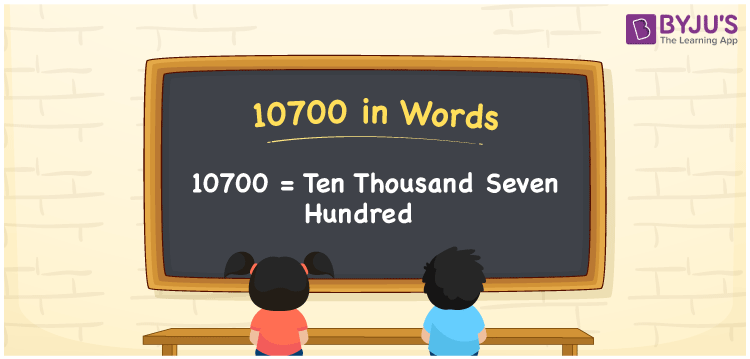# 10700 in Words

10700 in words can be written as Ten Thousand Seven Hundred. The fundamental concepts in Mathematics like counting or count can be learnt efficiently here. If you buy a scooter for Rs. 10700, then you can say that “I bought a scooter for Ten Thousand Seven Hundred Rupees”. To write numbers in words, the English alphabet is used. The numbers in words concept is explained here in a simple way to improve the conceptual knowledge of students. The number 10700 can be read as “Ten Thousand Seven Hundred” in English.

 10700 in words Ten Thousand Seven Hundred Ten Thousand Seven Hundred in Numbers 10700

## 10700 in English Words## How to Write 10700 in Words?

Students can learn about the expanded form and the place value chart of 10700. Five digits are present in the number 10700. With the help of the place value chart given below, students will be able to understand the concepts with ease.

 Ten Thousands Thousands Hundreds Tens Ones 1 0 7 0 0

10700 can be written in expanded form as:

1 x Ten Thousand + 0 x Thousand + 7 × Hundred + 0 × Ten + 0 × One

= 1 x 10000 + 0 x 1000 + 7 × 100 + 0 × 10 + 0 × 1

= 10000 + 700

= 10700

= Ten Thousand Seven Hundred

Hence, 10700 in words is written as Ten Thousand Seven Hundred.

10700 is a natural number that precedes 10701 and succeeds 10699.

10700 in words – Ten Thousand Seven Hundred

Is 10700 an odd number? – No

Is 10700 an even number? – Yes

Is 10700 a perfect square number? – No

Is 10700 a perfect cube number? – Yes

Is 10700 a prime number? – No

Is 10700 a composite number? – Yes

## Frequently Asked Questions on 10700 in Words

Q1

### How to write 10700 in words?

10700 can be written in words as “Ten Thousand Seven Hundred”.
Q2

### How to write Ten Thousand Seven Hundred in numbers?

Ten Thousand Seven Hundred in numbers can be written as 10700.
Q3

### Is 10700 an odd or even number?

10700 is an even number as it is completely divisible by 2.